Create a new printableMath Worksheets

Sample - Click above to make a new math worksheet (PDF).
 Name _____________________________Date ___________________
Length and Weight
Circle the object with the longest side.
 1 * This is a pre-made sheet.Use the link at the top of the page for a printable page.
2.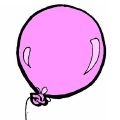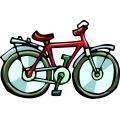3.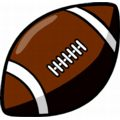4.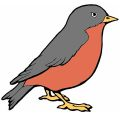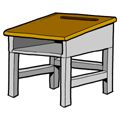Number the objects in order from shortest to longest. Compare using the longest side of each object.
Write 1 next to the object with the shortest length.  Write 3 next to the object with the longest length.

1.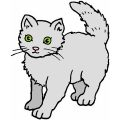______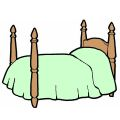______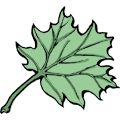______
2.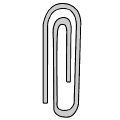______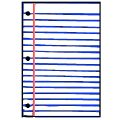______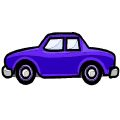______

Number the objects in order from longest to shortest. Compare using the longest side of each object.
Write 1 next to the object with the longest length.  Write 3 next to the object with the shortest length.

1.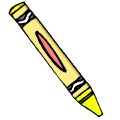____________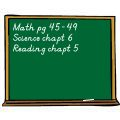______
2.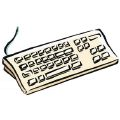______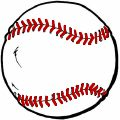____________

 Key #2
 aKey #2
Estimate how long the object is. Circle the answer that gives the best estimate.
1.4 inches 4 feet
2.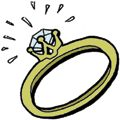1 inch 1 foot
3.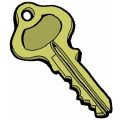3 inches 3 feet
4.1 inch 1 foot

Estimate how long the object is. Circle the answer that gives the best estimate.
1.3 centimeters 35 centimeters
2.2 meters 29 centimeters
3.30 centimeters 180 centimeters
4.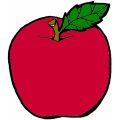10 centimeters 4 meters

Circle the object that is lighter.
1.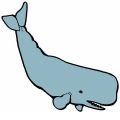2.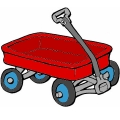3.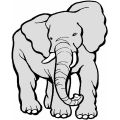4.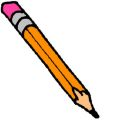Key #2
 aKey #2
Number the objects in order from lightest to heaviest.
Write 1 next to the lightest object.  Write 3 next to the heaviest object.

1.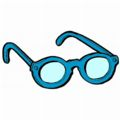______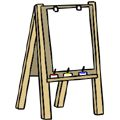____________
2.__________________

Estimate how much the object weighs. Circle the answer that gives the best estimate.
1.4 pounds 40 pounds
2.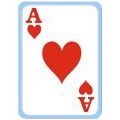1 pound 1 ounce
3.1 ounce 10 ounces
4.65,209 pounds 65,209 ounces

Estimate how much the object weighs. Circle the answer that gives the best estimate.
1.16 kilograms 1 gram
2.2 kilograms 2 grams
3.31 kilograms 31 grams
4.6,717 grams 6,717 kilograms